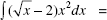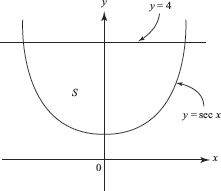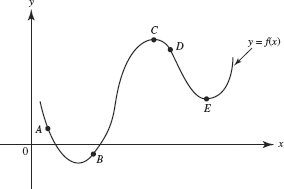# AP Calculus AB Practice Test 35

### Test Information8 questions24 minutes

Calculator Allowed

1.2.The region S in the figure shown above is bounded by y = sec x and y = 4. What is the volume of the solid formed when S is rotated about the x-axis?

3.At which point on the graph of y = f (x) shown above is f (x) < 0 and f (x) > 0?

4. Let f (x) = x5 + 1, and let g be the inverse function of f. What is the value of g (0)?

5. The hypotenuse AB of a right triangle ABC is 5 feet, and one leg, AC, is decreasing at the rate of 2 feet per second. The rate, in square feet per second,at which the area is changing when AC = 3 is

6. At how many points on the interval [0,π] does f (x) = 2 sin x + sin 4x satisfy the Mean Value Theorem?

7. If the radius r of a sphere is increasing at a constant rate, then the rate of increase of the volumeof the sphere is

8. The rate at which a purification process can remove contaminants from a tank of wateris proportional to the amount of contaminant remaining. If 20% of the contaminantcan be removed during the first minute of the process and 98% must be removed to makethe water safe, approximately how long will the decontamination process take?